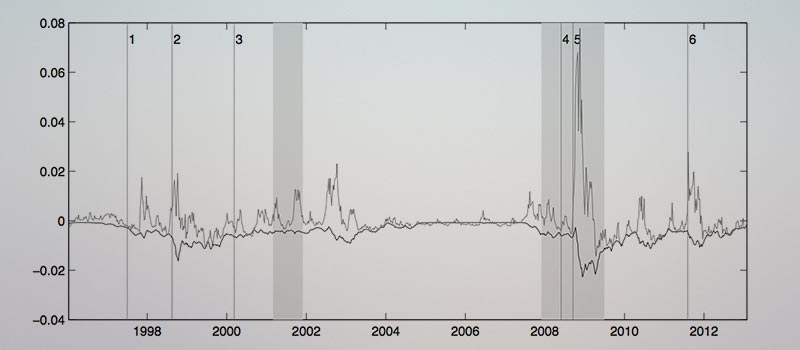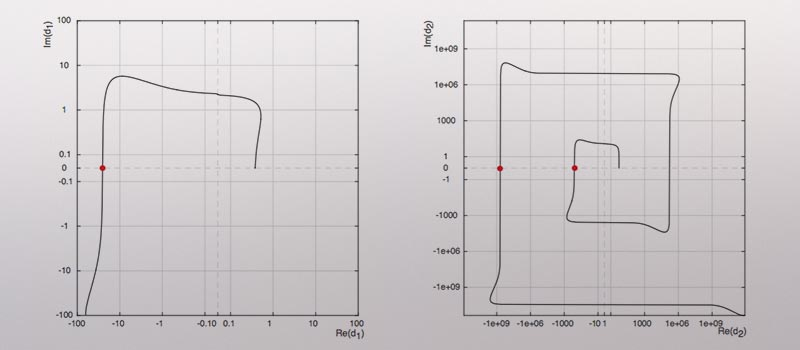## The price of the Smile and Variance Risk PremiaIn a tractable stochastic volatility model, we identify the price of the smile as the price of the unspanned risks traded in SPX option markets. The price of the smile reflects two persistent volatility and skewness risk factors, which imply a downward sloping term structure of low-frequency variance risk premia in normal times. In periods of distress, the term structure is upward sloping and dominated by a high-frequency premium for jump variance. This dichotomy is consistent with the puzzling skew sensitivities of option markets with credit- constrained intermediaries and it builds a challenge for many reduced-form and structural models of stochastic volatility.

• Option Pricing
• Factor Models

## Eliciting a Smile

Numerical Methods for Option Pricing with Matrix Affine Jump DiffusionsI present a framework for the efficient and stable estimation of matrix affine jump diffusion (MAJD) volatility models using transform methods. Due to its matrix nature, there are several computational challenges for evaluating the Laplace transform of MAJD models: (i) it contains a multivalued complex logarithm, causing a potentially large bias in option prices, (ii) it is computationally more costly by two orders of magnitude compared to similar scalar models, and (iii) it contains a numerically unstable matrix inversion. To solve these problems, I formulate the matrix rotation count algorithm for the complex logarithm and I propose a volatility-independent approximation of the integration limits for the Cosine Fourier inversion. The latter forms the basis of a fast estimation scheme via a separation of the state- and parameter-dependent parts of the Laplace transform. The results can also be applied to scalar multivariate affine models, as they are nested in the MAJD class.

• Numerics
• Rotation Count
• COS-FFT

## Three Make a Dynamic Smile

Unspanned Skewness and Interacting Volatility Components in Option ValuationWe study a new class of three-factor affine option pricing models with interdependent volatility dynamics and a stochastic skewness component unrelated to volatility shocks. These properties are useful in order (i) to model a term structure of implied volatility skews more consistent with the data and (ii) to capture comovements of short and long term skews largely unrelated to the volatility dynamics. We estimate our models using about fourteen years of S&P 500 index option data and find that on average they improve the out-of-sample pricing accuracy of benchmark two- and three-factor affine models by 20%. Using an appropriate decomposition of volatility and skewness, highlighting the main directions of improvements produced by our setting, we show that the enhanced fit results from an improved modeling of the term structure of implied-volatility skews. The largest pricing improvements tend to concentrate during periods of financial crises or market distress, suggesting volatility- unrelated skewness as a potentially useful reduced-form risk factor for reproducing some of the crisis-related dynamics of index option smiles.

• Option Pricing
• Matrix Affine Processes
• Unspanned Skewness